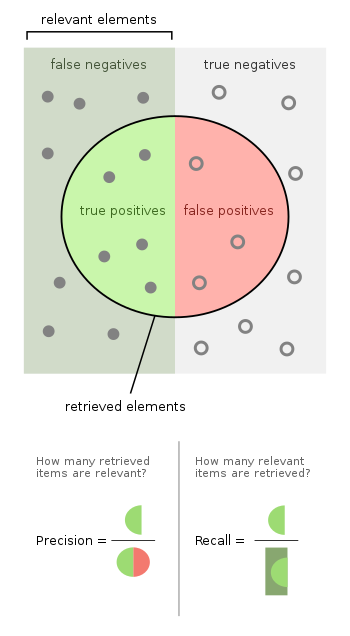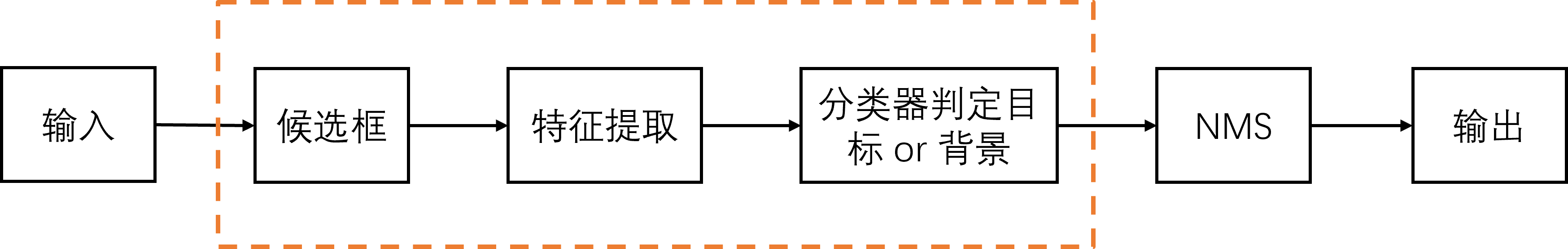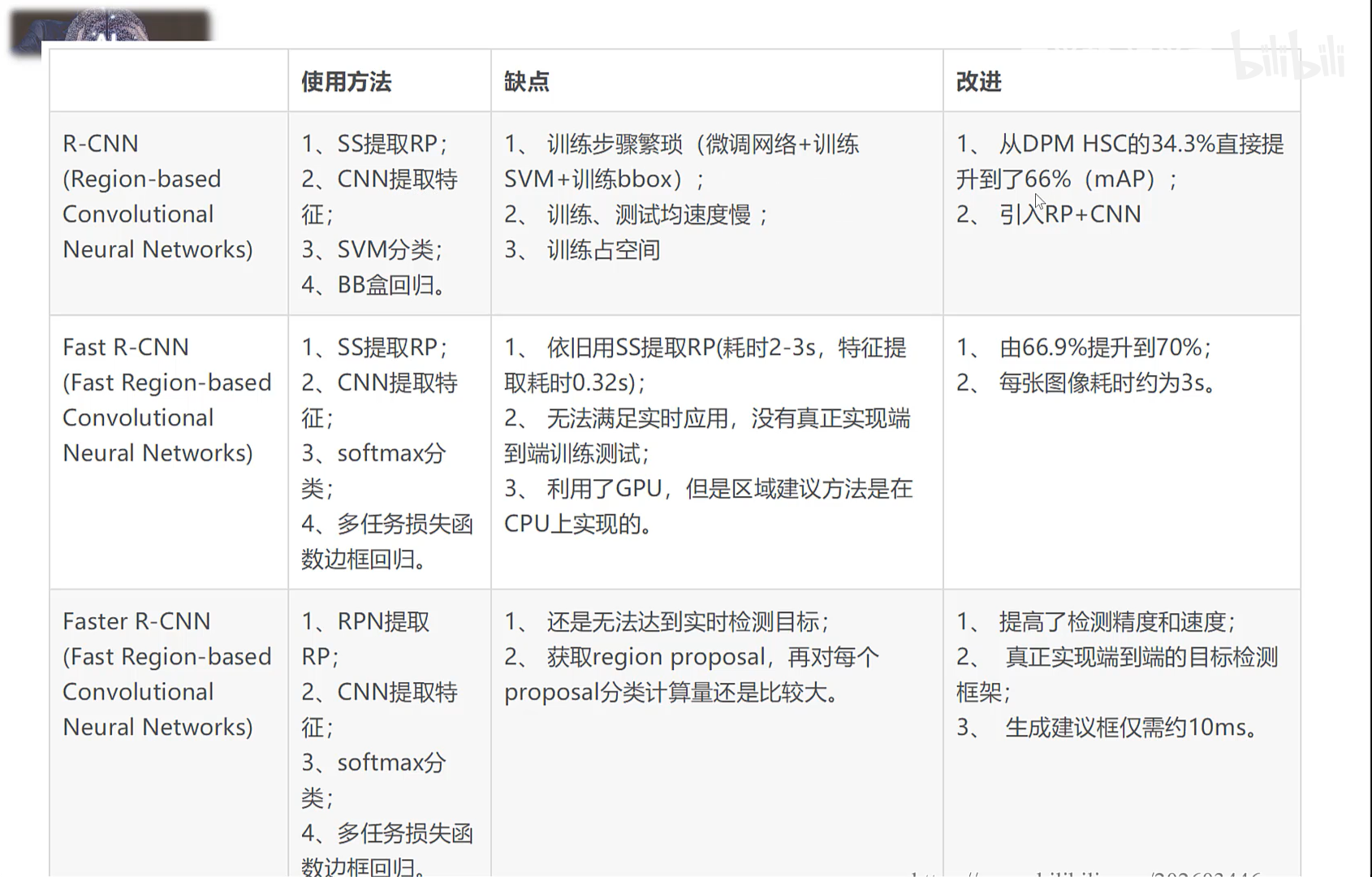# 目标检测概述

## 1. 简介

• 传统的目标检测算法
• Cascade + HOG / DPM + Haar / SVM
• 候选窗 + 深度学习分类
• 提取候选区域 + 深度深度习方法分类
• R-CNN（Selective Search + CNN + SVM）SPP-Net（ROI Pooling）Fast R-CNN（Selective Search + CNN + ROI）Faster R-CNN（RPN + CNN + ROI）R-FCN 等系列方法
• 基于深度学习的回归方法
• YOLO / SSD / DenseBox 等方法
• 结合 RNN 算法的 RRC detection；结合 DPM 的 Deformable CNN 等

• 单阶段 One-stage：YOLO 系列

## 2. 相关指标

### 2.1 F1-Score

• TP：True Positive，正样本被正确分为了正类；
• TN：True Negative，负样本被正确分为了负类；
• FP：False Positive，负样本被错误分为了正类；
• FN：False Negative，正样本被错误分为了负类。$\mathrm{Accuracy} = \frac{TP + TN}{TP + FN + FP + TN}$

$\mathrm{Precision} = \frac{TP}{TP + FP}$

$\mathrm{Recall} = \frac{TP}{TP + FN}$

F1-Score 的计算公式如下（即精确率和召回率的调和平均数）：

$\mathrm{F1} = \frac{2 \times \mathrm{Precision} \times \mathrm{Recall}}{\mathrm{Precision} + \mathrm{Recall}}$

PR 曲线是指查准率-查全率曲线，横坐标为查全率，纵坐标为查准率。以二分类模型为例，其绘制是根据所选取的分类阈值来得到的。不同的分类阈值，会产生不同的查准率-查全率数据对。

### 2.2 IoU

IoU 也即交并比，是指模型所预测的检测框和真实的检测框的交集和并集之间的比例。

$\mathrm{IoU} = \frac{\mathrm{Intersection}}{\mathrm{Union}}$

$x_1 = \max(x_{1, 1}, x_{2, 1}), x_2 = \min(x_{1, 2}, x_{2, 2}) \\ y_1 = \max(y_{1, 1}, y_{2, 1}), y_2 = \min(y_{1, 2}, y_{2, 2}) \\ w = \max(0, x_2 - x_1) \\ h = \max(0, y_2 - y_1) \\ \mathrm{Intersection} = w \cdot h \\ \mathrm{Union} = (x_{1, 2} - x_{1, 1}) \cdot (y_{1, 2} - y_{1, 1}) + (x_{2, 2} - x_{2, 1}) \cdot (y_{2, 2} - y_{2, 1}) - \mathrm{Intersection}$

### 2.3 ROC & AUC

$\mathrm{Sensitivity} = \frac{TP}{TP + FN}$

$\mathrm{Specificity} = \frac{TN}{FP + TN}$

$TPR = \mathrm{Sensitivity} = \frac{TP}{TP + FN} \\ FDR = 1 - \mathrm{Specificity} = \frac{FP}{FP + TN}$

TPR 只关注系统的正样本部分中有多少是被真正覆盖的，而无关系统中的负样本部分；FDR 则相反，只关注系统的负样本中有多少是被错误覆盖的，而无关系统中的正样本部分。

**ROC **曲线是指 TPR-FPR 曲线，横坐标为 FPR，纵坐标为 TPR。与 PR 曲线类似，ROC 曲线也是通过遍历所有阈值来绘制整条曲线的。

ROC 曲线无视样本不平衡的问题。因为 TPR 和 FPR 都是只针对实际的某类样本，因而不受样本不平衡的影响。

AUC 曲线是指 ROC 曲线下方的面积。AUC 最差时对应随机判断，此时 AUC 取值为 $0.5$。ROC 曲线越陡越好，AUC 的值是介于 $0.5$$1$ 之间的。

### 2.3 AP & mAP

AP(Average Precision) 是指单类标签平均的精确率，简单来说便是 PR 曲线下方的面积。mAP(Average Precision) 则是对所有类的 AP 值求平均。

## 3. 传统目标检测

### 3.1 基本流程• 候选框的提取：候选窗的提取通常采用滑动窗口的方法（时耗较大）
• 特征提取：基于颜色、基于纹理、基于形状的方法，以及一些中层次或高层次语义特征的方法。目标检测算法中通常使用的方法集中在低层和中层。
• 目标判别：对候选区域提取出的特征进行分类判定。单类别目标检测：区分背景和目标；多分类问题：区分当前窗口中对像的类别。
• NMS：解决候选框重叠问题，NMS 对候选框进行合并。

### 3.2 候选框

• 滑窗法：使用预先定义好的不同大小的窗口，穷举式滑动遍历整张图像，并将每次窗口对应的 Patch 输入分类器，判定是否有目标。

• 选择性搜索：自下向上，从像素级开始，根据颜色/纹理/大小等相似信息，不断往上合并，最后得到各个目标的大致窗口。选择性搜索速度快，召回率高。

具体是如何合并的呢？

使用基于论文《Efficient Graph-Based Image Segmentation》中的方法。

CNN 网络，比如 VGG-16

### 3.4 分类

Bound Box Regression 是如何做的？

## 4. R-CNN 系列### 4.1 R-CNN

R-CNN 算法可分为四个步骤：

• 一张图像生成 1K ～ 2K 个候选区域（使用选择性搜索）；
• 对每个候选区域，使用深度网络提取特征；
• 特征送入每一类的 SVM 分类器，判别是否属于该类；
• 使用回归器精细修正候选框的位置。

R-CNN 算法存在的问题：

• 测试速度慢，测试一张图需要 CPU 53 秒。用选择性搜索算法提取候选框用时约 2 秒，一张图像内候选框之间存在大量重叠，提取特征操作冗余。
• 训练速度慢，过程及其繁琐。
• 训练所需空间大，对于 SVM 和 BBox 回归训练，需要从每个图像中的每个目标候选框提取特征，并写入磁盘。对于非常深的网络，如 VGG16，从 VOCO7 训练集上的 5K 图像上提取特征需要数百 GB 的存储空间。

SPP 层是如何处理多个目标的？

SPP-Net 的缺点。

### 4.3 Fast R-CNN

Fast R-CNN 算法流程可分为三个步骤：

• 一张图像生成 1K ～ 2K 个候选区域（使用选择性搜索）；
• 将图像输入深度网络得到相应的特征图，将 SS 算法生成的候选框投影到特征图上获得相应的特征矩阵；
• 将每个特征矩阵通过 ROI Pooling 层缩放到 $7 \times 7$ 大小的特征图，接着将特征图展平通过一系列全连接层得到预测结果。

FPN 网络结构。

### 4.4 Faster R-CNN

Faster R-CNN 如何将分类和 BBR 一起多损失函数训练的？Multi-task Loss 是什么？RPN 结构是什么？

Faster R-CNN 算法流程可分为三个步骤：

• 将图像输入网络得到相应的特征图；
• 使用 RPN 结构生成候选框，将 RPN 生成的候选框投影到特征图上获得相应的特征矩阵；
• 将每个特征矩阵通过 ROI Pooling 层缩放到 $7 \times 7$ 大小的特征图，接着将特征图展平通过一系列全连接层得到预测结果。

Faster